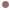Autres Articles de :
Lorenz-like systems

# 1992 A model for weakly nonlinear double convection

Christophe LETELLIER
10/05/2009
Alastair RucklidgeIn certain parameter regimes, it is possible to derive third-order sets of ordinary differential equations that are asymptotically exact descriptions of weakly nonlinear double convection and that produce chaotic behaviours. Cases of two-dimensional convection in a horizontal layer of Boussinesq fluid with lateral constraints were considered by Alastair Ruckldige. When the convection takes place in a fluid layer rotating uniformly about a vertical axis and in the limit of tall thin rolls, convection in an imposed vertical magnetic field and convection in a rotating fluid layer are both modelled by a new third-order set of ordinary differential equations, which produces chaotic solutions that are created in a homoclinic explosion, in the same manner as the chaotic solutions to the Lorenz equations. Unlike the Lorenz equations, however, this model provides an accurate description of convection in the parameter regime where the chaotic solutions appear.The system

The set of three ordinary differential equationsproduces a chaotic attractor (Fig. 1) which is topologically equivalent to the Lorenz attractor. This is obtained with parameter valuesand.Fig. 1 : Chaotic "Lorenz" attractor solution to the Rucklidge system.

When the parameter values are replaced withand, the attractor solution to the Rucklidge system is topologically equivalent to the "Burke and Shaw" attractor (Fig. 2).Fig. 2 : Chaotic "Burke and Shaw" attractor solution to the Rucklidge system.

 A. M. Rucklidge, Chaos in models of double convection, Journal of Fluid Mechanics, 237, 209-229, 1992.